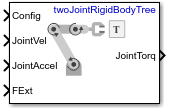# Inverse Dynamics

Required joint torques for given motion

• Library:
• Robotics System Toolbox / Manipulator Algorithms

•## Description

The Inverse Dynamics block returns the joint torques required for the robot to maintain the specified robot state. To get the required joint torques, specify the robot configuration (joint positions), joint velocities, joint accelerations, and external forces.

## Ports

### Input

expand all

Robot configuration, specified as a vector of positions for all nonfixed joints in the robot model, as set by the Rigid body tree parameter. You can also generate this vector for a complex robot using the `homeConfiguration` or `randomConfiguration` functions inside a Constant or MATLAB Function block.

Joint velocities, specified as a vector. The number of joint velocities is equal to the degrees of freedom (number of nonfixed joints) of the robot.

Joint accelerations, specified as a vector. The number of joint accelerations is equal to the degrees of freedom of the robot.

External force matrix, specified as a 6-by-n matrix, where n is the number of bodies in the robot model. The matrix contains nonzero values in the rows corresponding to specific bodies. Each row is a vector of applied forces and torques that act as a wrench for that specific body. Generate this matrix using `externalForce` with a MATLAB Function block

### Output

expand all

Joint torques, returned as a vector. Each element corresponds to a torque applied to a specific joint. The number of joint torques is equal to the degrees of freedom (number of nonfixed joints) of the robot.

## Parameters

expand all

Robot model, specified as a `rigidBodyTree` object. You can also import a robot model from an URDF (Unified Robot Description Formation) file using `importrobot`.

The default robot model, `twoJointRigidBodyTree`, is a robot with revolute joints and two degrees of freedom.

• `Interpreted execution` — Simulate model using the MATLAB® interpreter. This option shortens startup time but has a slower simulation speed than ```Code generation```. In this mode, you can debug the source code of the block.

• `Code generation` — Simulate model using generated C code. The first time you run a simulation, Simulink® generates C code for the block. The C code is reused for subsequent simulations, as long as the model does not change. This option requires additional startup time, but the speed of the subsequent simulations is comparable to ```Interpreted execution```.

Tunable: No

## Extended Capabilities

### C/C++ Code GenerationGenerate C and C++ code using Simulink® Coder™.

Introduced in R2018a